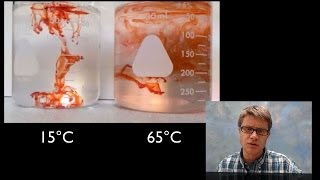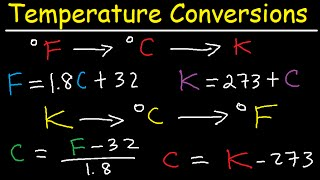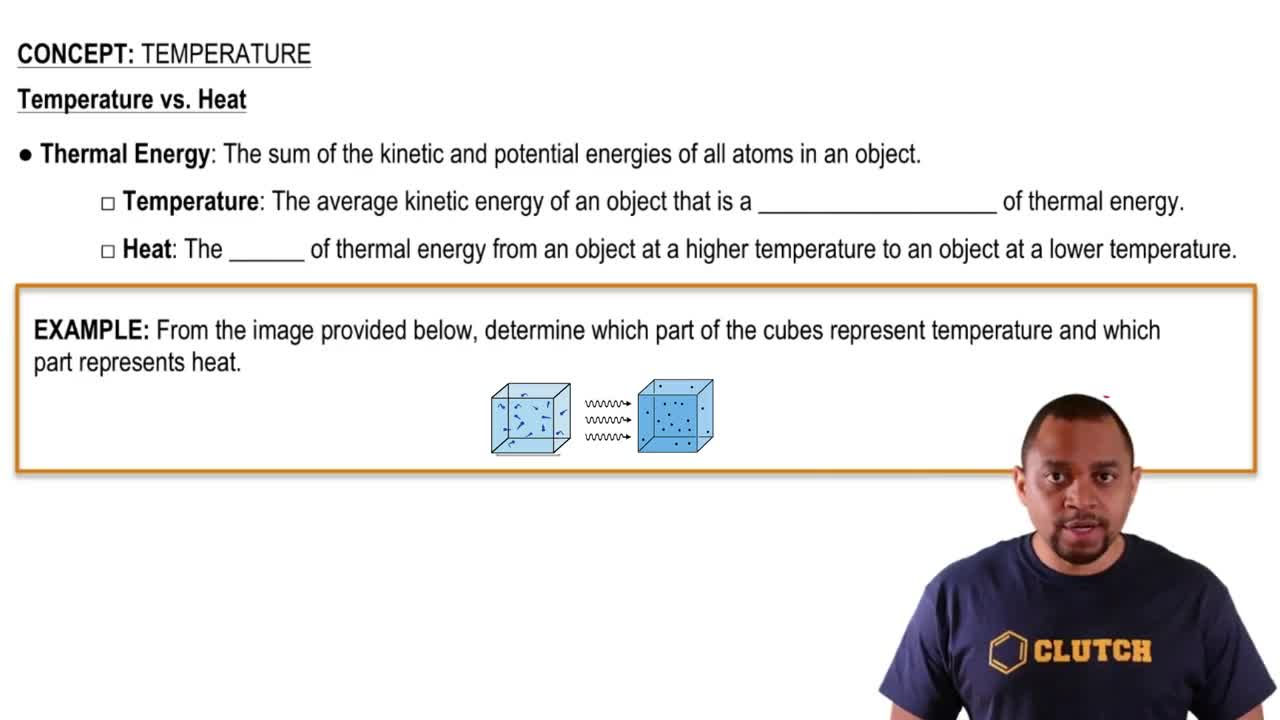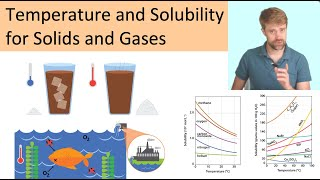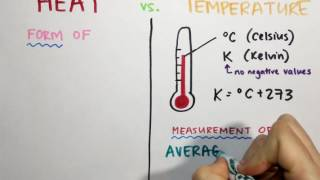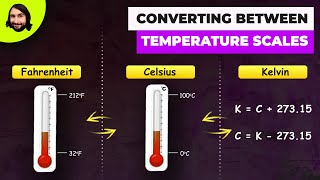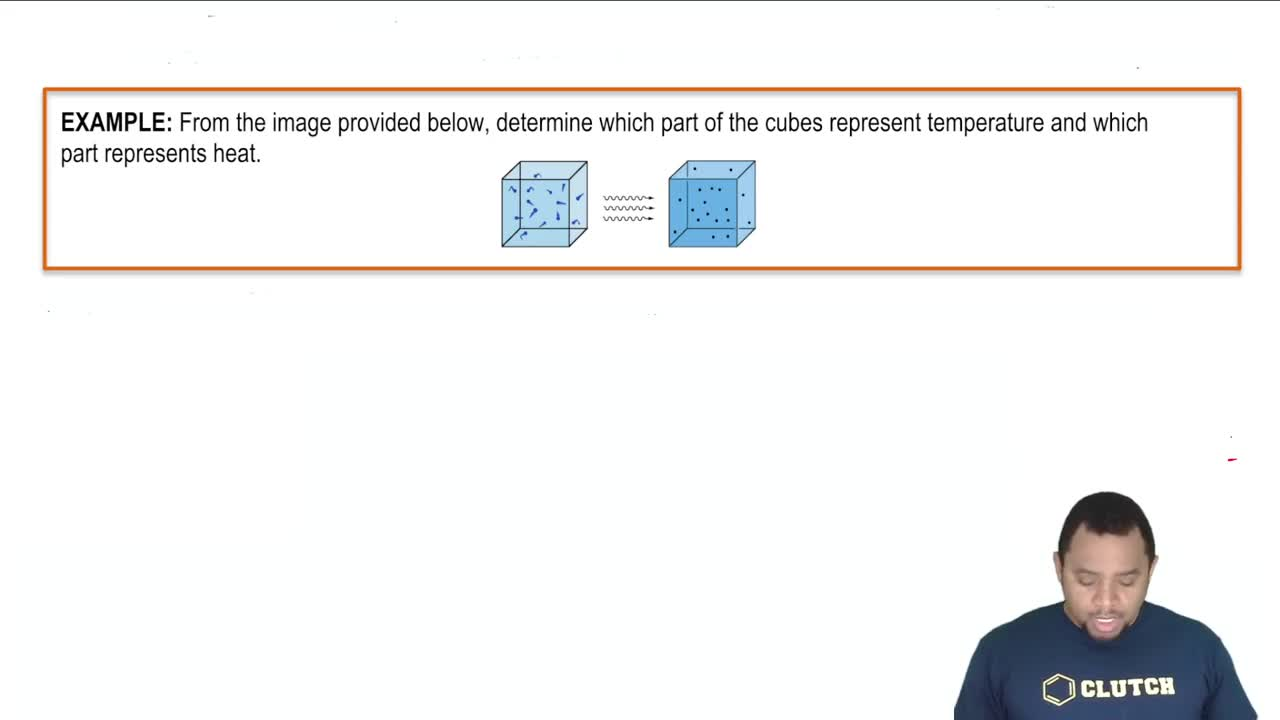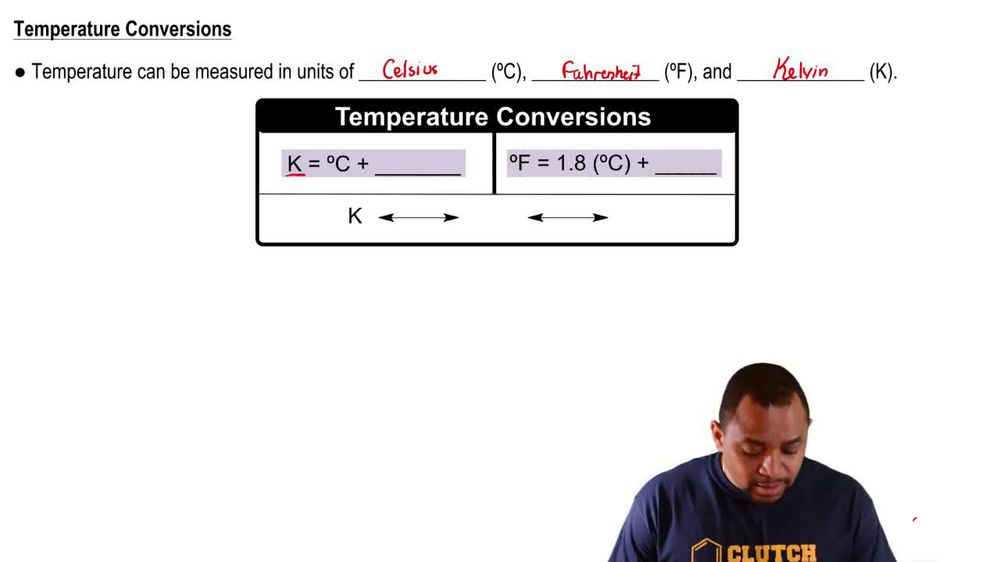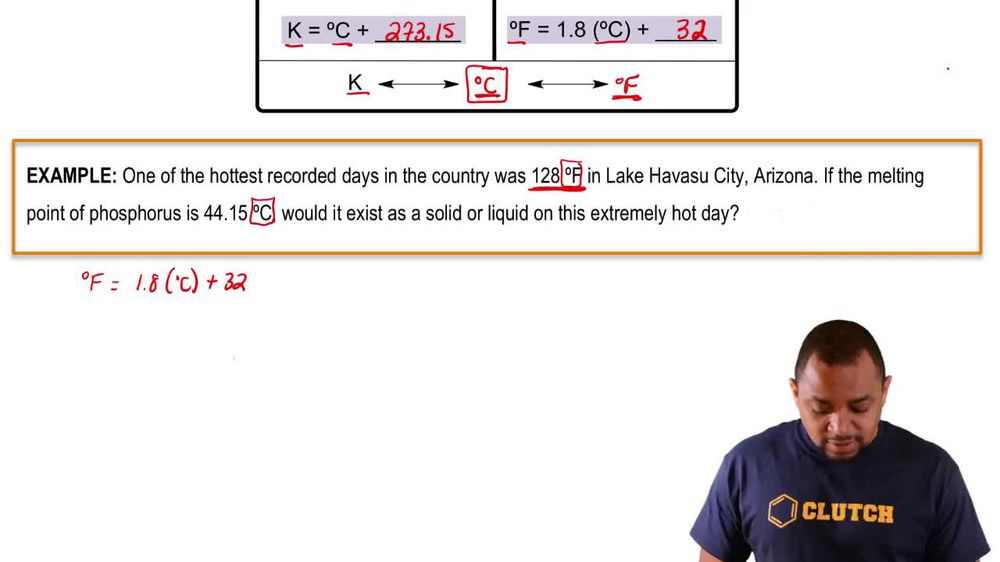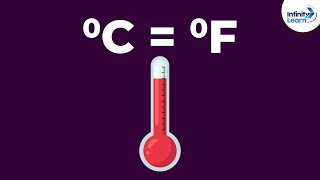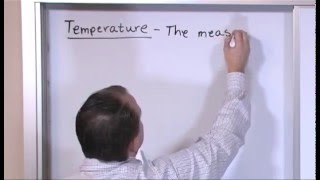Start typing, then use the up and down arrows to select an option from the list.
1. 1. Intro to General Chemistry2. Temperature
Problem

# Suppose you were dissatisfied with both Celsius and Fahrenheit units and wanted to design your own temperature scale based on ethyl alcohol (ethanol). On the Celsius scale, ethanol has a melting point of -117.3 °C and a boiling point of 78.5 °C, but on your new scale calibrated in units of degrees ethanol, °E, you define ethanol to melt at 0 °E and boil at 200 °E. (d) What is normal human body temperature (98.6 °F) on the ethanol scale?

Relevant Solution3m
Play a video:
Hello everyone today you're being given the falling problem suppose you wanted to create your own temperature scale based on pro pill, alcohol or propane all. Since you were unsatisfied with both the Celsius and Fahrenheit units the melting point and boiling point of propane on the Celsius scale or negative 1 26 degrees Celsius and 97.2 degrees Celsius respectively. In the new scale you define the melting point in boiling point of propane all at zero degrees P and 150° propane all respectively. What is your standard temperature? 59°F in degrees propane all or degrees. So first we want to find the boiling point and the melting point both in terms of degrees Fahrenheit. So we have here are propane all boiling point and our propane all melting point. And so it was initially established on the Celsius scale that the boiling point Was 97.2°C and the melting point was 1 26°C. When we go ahead and convert that to Fahrenheit, we get so forget the boiling point. That's going to be equal to 97.2 degrees Celsius times 9/5 plus 32 to give us 206.96 degrees Fahrenheit. So 206.96 degrees Fahrenheit and then we have our melting point which is going to be negative 1 26 degrees Celsius times 9/5 plus which would give us negative 1 94.8. So we're going to fill that in here -194.8°F. And so we must know, convert Our 59°F and degrees propofol. So for degrees of propane all, we're going to take our 150 degrees probe and all that we initially had and we're going to divide that by our degrees in Fahrenheit for boiling point, which was 206.96 degrees Fahrenheit, we're gonna subtract that by 1 94.8 or the melting point. And they were going to multiply this By our 59°F Plus our 1 94.8° as well and fair and higher for the melting point. So when we do this, we get 94.76° proposal as our final answer. Overall, I hope this helped. And until next time.